# Introduction to Javascript HTML5 Canvas API

#### View more Tutorials: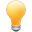Follow us on our fanpages to receive notifications every time there are new articles.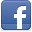Facebook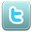Twitter

## 1- HTML5 Canvas

Before HTML5 was created, when web developers wanted to use graphics or animation on the website, a product of the thirt party such as Flash, Java Applet had to be embedded. Although this may solve the problem, the website becomes heavier and there is something that has not satisfied you.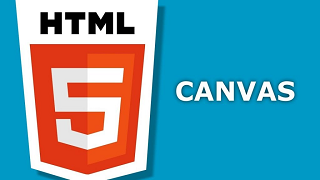When HTML5 was launched, it introduced people to many new features and tags including the <canvas> element. The <canvas> element creates a rectangular area and you need to use Javascript to draw on that area.
Canvas is available as an API and it is supported by modern browsers. Moreover, it is compatible with multiple platforms. Therefore, you can create applications once and deploy to many different platforms - PCs or mobile devices.
As a result, you can create a game like this with Javascript only: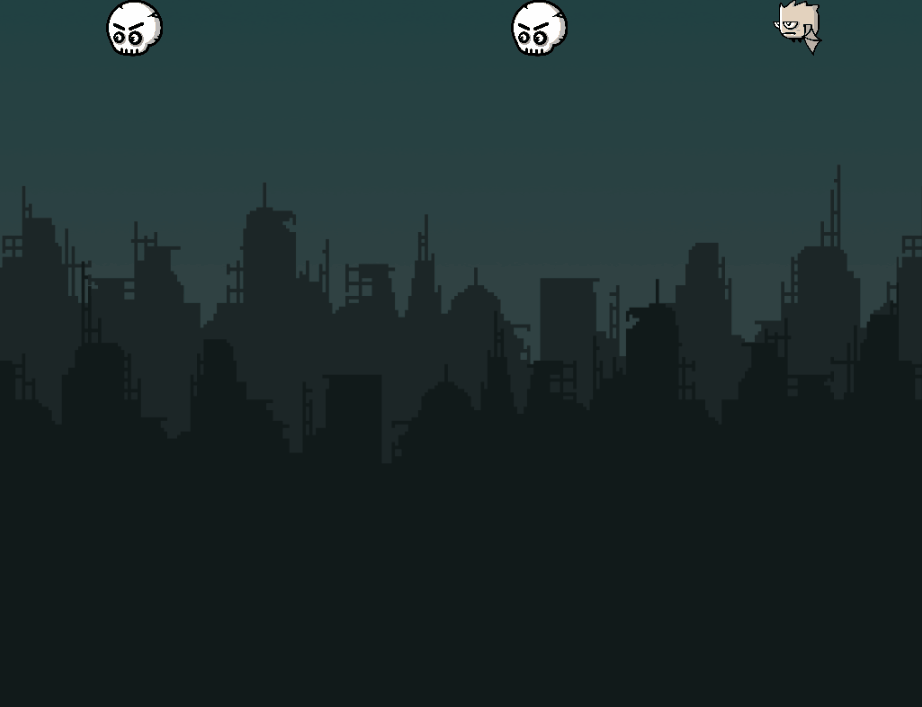Browsers support the <canvas> ​​​​​​​element: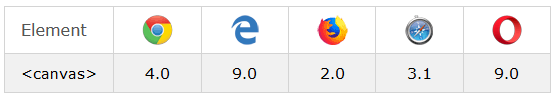## 2- Some examples of Canvas:

In this section, I'm going to introduce you to several examples using HTML5 Canvas, the main purpose of which is to help you visualize what you can do with HTML5 Canvas.
<canvas>
By default, the  <canvas> element creates a rectangular area without border and content. You can set the width and height for the <canvas> by using the width and height attributes. If the width and height attributes are not specified, they will have a default value of 300px and 150px respectively.
first-canvas-example.html
``````
<!DOCTYPE html>
<html>
<title>Canvas</title>
<meta charset="UTF-8">
<body>
<h1>HTML5 Canvas</h1>
<canvas style="border:1px solid black" width=280 height=150>
Your browser does not support the HTML5 Canvas.
</canvas>
</body>
</html>
``````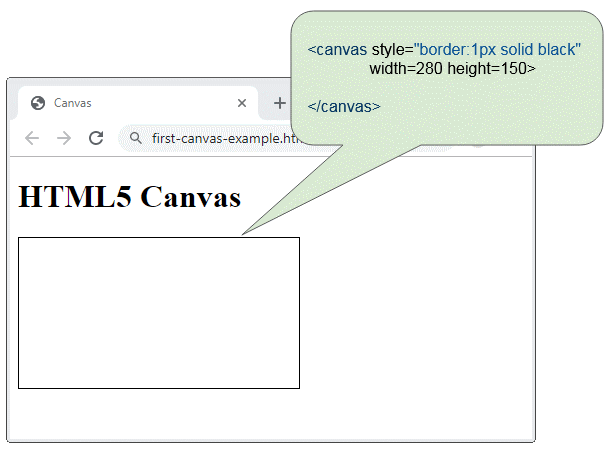An example of drawing a circle on Canvas:
``````
function drawCircle() {
var c = document.getElementById("myCanvas");
var ctx = c.getContext("2d");
ctx.beginPath();
ctx.arc(95, 50, 40, 0, 2 * Math.PI);
ctx.stroke();
}
``````
See full source code of the example here:
draw-circle-example.html
``````
<!DOCTYPE html>
<html>
<title>Canvas</title>
<meta charset="UTF-8">
<script>
function drawCircle()  {
var c = document.getElementById("myCanvas");
var ctx = c.getContext("2d");
ctx.beginPath();
ctx.arc(95, 50, 40, 0, 2 * Math.PI);
ctx.stroke();
}
</script>
<body>
<h2>Draw Circle</h2>
<button onclick="drawCircle()">Draw Circle</button>
<a href="">Reset</a>
<br/><br/>
<canvas id="myCanvas" style="border:1px solid black" width=280 height=150>
Your browser does not support the HTML5 Canvas.
</canvas>
</body>
</html>
``````
HTML 5 Clock
The example below uses Javascript and Canvas to create a clock that displays the current time. This shows that the Canvas API can create animation effects.
clock.js
``````
function drawClock() {
var canvas = document.getElementById('myClock');
if (canvas.getContext) {
var c2d = canvas.getContext('2d');
c2d.clearRect(0, 0, 300, 300);
c2d.font = "Bold 20px Arial";
c2d.textBaseline = "middle";
c2d.textAlign = "center";
c2d.lineWidth = 1;
c2d.save();
//Outer bezel
c2d.lineWidth = 10;
c2d.beginPath();
c2d.arc(150, 150, 138, 0, Math.PI * 2, true);
c2d.stroke();
//Inner bezel
c2d.restore();
c2d.lineWidth = 10;
c2d.beginPath();
c2d.arc(150, 150, 129, 0, Math.PI * 2, true);
c2d.stroke();
c2d.strokeStyle = "#222";
c2d.save();
c2d.translate(150, 150);
//Markings/Numerals
for (i = 1; i <= 60; i++) {
ang = Math.PI / 30 * i;
sang = Math.sin(ang);
cang = Math.cos(ang);
//If modulus of divide by 5 is zero then draw an hour marker/numeral
if (i % 5 == 0) {
c2d.lineWidth = 8;
sx = sang * 95;
sy = cang * -95;
ex = sang * 120;
ey = cang * -120;
nx = sang * 80;
ny = cang * -80;
c2d.fillText(i / 5, nx, ny);
//Else this is a minute marker
} else {
c2d.lineWidth = 2;
sx = sang * 110;
sy = cang * 110;
ex = sang * 120;
ey = cang * 120;
}
c2d.beginPath();
c2d.moveTo(sx, sy);
c2d.lineTo(ex, ey);
c2d.stroke();
}
//Fetch the current time
var ampm = "AM";
var now = new Date();
var hrs = now.getHours();
var min = now.getMinutes();
var sec = now.getSeconds();
c2d.strokeStyle = "#000";
//Draw AM/PM indicator
if (hrs >= 12) ampm = "PM";
c2d.lineWidth = 1;
c2d.strokeRect(21, -14, 44, 27);
c2d.fillText(ampm, 43, 0);

c2d.lineWidth = 6;
c2d.save();
//Draw clock pointers but this time rotate the canvas rather than
//calculate x/y start/end positions.
//
//Draw hour hand
c2d.rotate(Math.PI / 6 * (hrs + (min / 60) + (sec / 3600)));
c2d.beginPath();
c2d.moveTo(0, 10);
c2d.lineTo(0, -60);
c2d.stroke();
c2d.restore();
c2d.save();
//Draw minute hand
c2d.rotate(Math.PI / 30 * (min + (sec / 60)));
c2d.beginPath();
c2d.moveTo(0, 20);
c2d.lineTo(0, -110);
c2d.stroke();
c2d.restore();
c2d.save();
//Draw second hand
c2d.rotate(Math.PI / 30 * sec);
c2d.strokeStyle = "#E33";
c2d.beginPath();
c2d.moveTo(0, 20);
c2d.lineTo(0, -110);
c2d.stroke();
c2d.restore();

//Additional restore to go back to state before translate
//Alternative would be to simply reverse the original translate
c2d.restore();
setTimeout(drawClock, 1000);
}
}
``````
clock.html
``````
<!DOCTYPE html>
<html>
<title>Clock</title>
<meta charset="UTF-8">
<script src="clock.js"></script>